# SSAT Upper Level Math : How to find consecutive integers

## Example Questions

### Example Question #1 : Sequences And Series

Three consecutive even integers have sum 924. What is the product of the least and greatest of the three?

Possible Answers: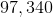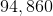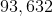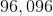No three consecutive even integers have sum 924.

Correct answer:Explanation:

Let the middle integer of the three be. The three integers are therefore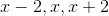, and they can be found using the equation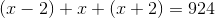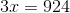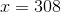The three even integers are therefore 306, 308, and 310, and the product of the least and greatest of these is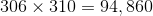### Example Question #2 : Sequences And Series

Three consecutive odd integers have sum 537. What is the product of the least and greatest of the three?

Possible Answers: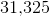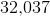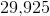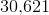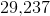Correct answer:Explanation:

Let the middle integer of the three be. The three integers are therefore, and they can be found using the equation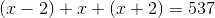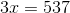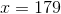The three integers are 177, 179, and 181, and the product of the least and greatest is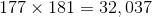### Example Question #3 : Sequences And Series

Four consecutive integers have sum 3,350. What is the product of the middle two?

Possible Answers: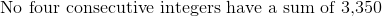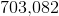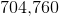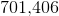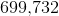Correct answer:Explanation:

Call the least of the four integers. The four integers are therefore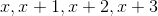,

and they can be found using the equation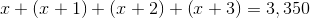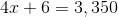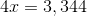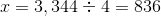The integers are 836, 837, 838, 839.

To get the correct response, multiply: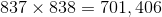### Example Question #1 : Consecutive Integers

Three consecutive integers have sum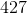. What is their product?

Possible Answers: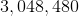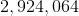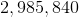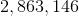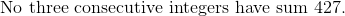Correct answer:Explanation:

Let the middle integer of the three be. The three integers are therefore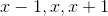, and they can be found using the equation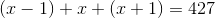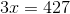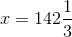This contradicts the condition that the numbers are integers. Therefore, three integers satisfying the given conditions cannot exist.

### Example Question #1 : Consecutive Integers

Three consecutive integers have a sum of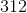. What is their product?

Possible Answers: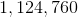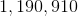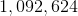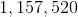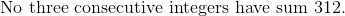Correct answer:Explanation:

Let the middle integer of the three be. The three integers are therefore, and they can be found using the equation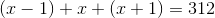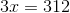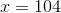The integers are therefore 103, 104, 105. The correct response is their product, which is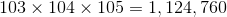### Example Question #1 : Consecutive Integers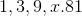What is the value ofis this sequence?

Possible Answers: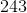Correct answer:Explanation:

This is a geometric sequence since the pattern of the sequence is through multiplication.

You have to multiple each value byto get the next one.

The value beforeisso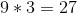.

### Example Question #2 : Consecutive Integers

Which of the following can be the sum of four consecutive positive integers?

Possible Answers: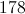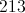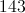None of the other answers are correct.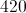Correct answer:Explanation:

Let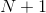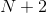, and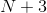be the four consecutive integers. Then their sum would be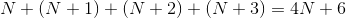In other words, if 6 were to be subtracted from their sum, the difference would be a multiple of 4. Therefore, we subtract 6 from each of the choices and see if any of the resulting differences are multiples of 4.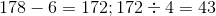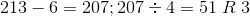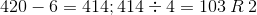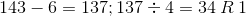Since this only happens in the case of 178, this is the only number of the four that can be a sum of four consecutive integers: 43, 44, 45, 46.

### All SSAT Upper Level Math Resources